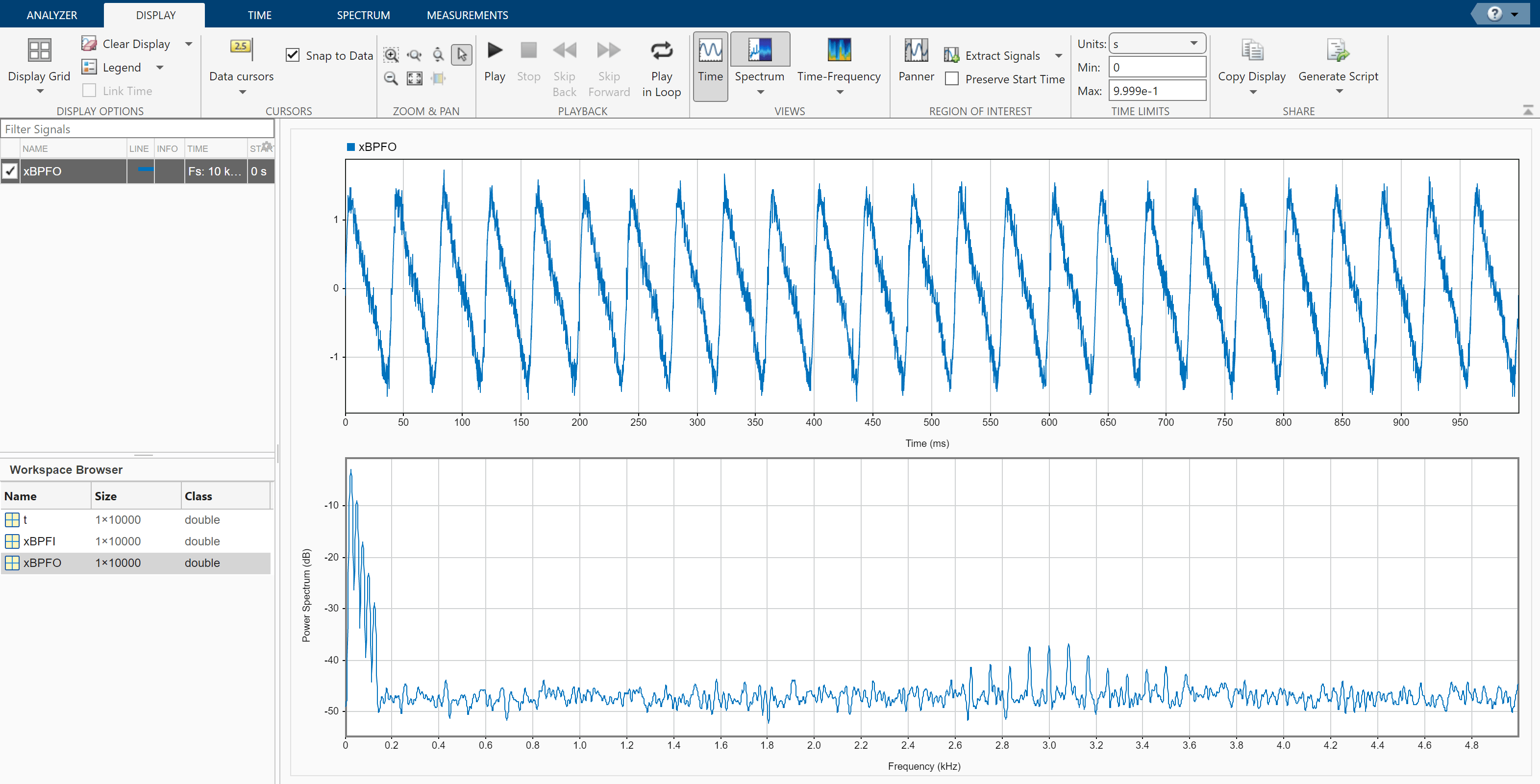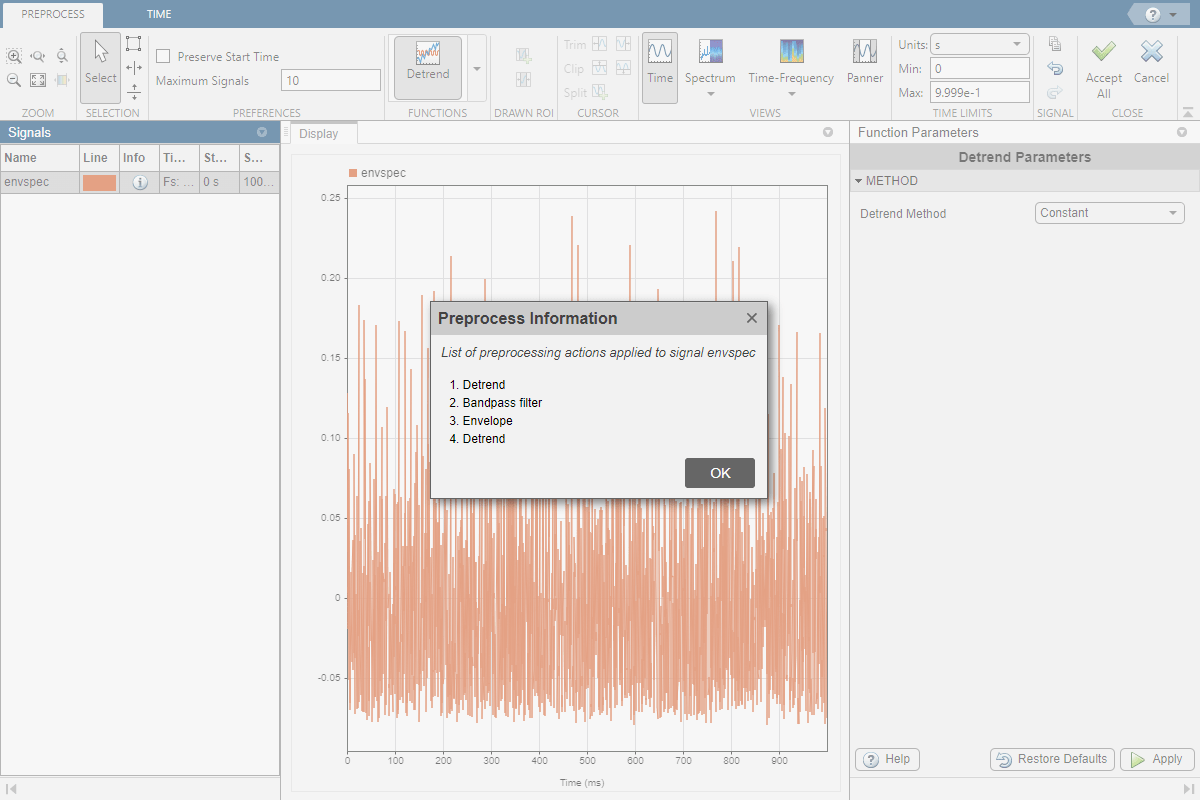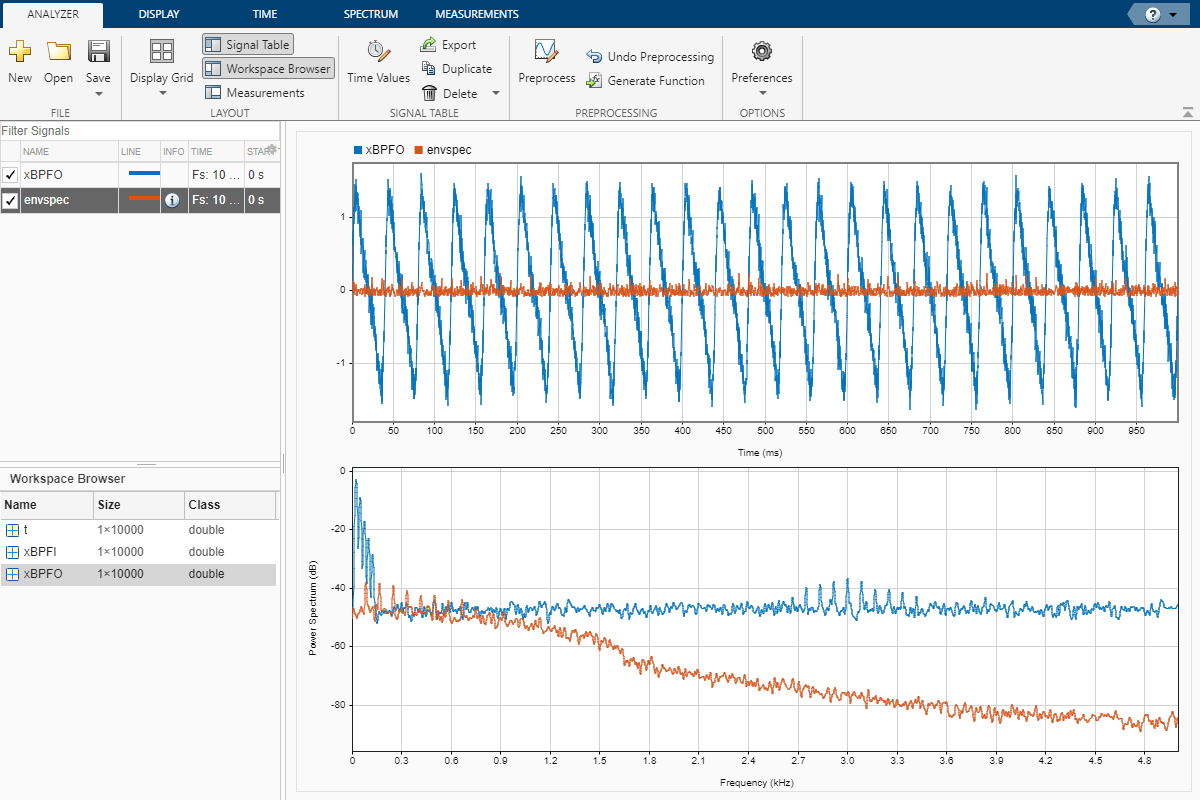Main Content

# Compute Envelope Spectrum of Vibration Signal

Use Signal Analyzer to compute the envelope spectrum of a bearing vibration signal and look for defects. Generate MATLAB® scripts and functions to automate the analysis.

### Generate Bearing Vibration Data

A bearing with the dimensions shown in the figure is driven at ${\mathit{f}}_{0}=25$ cycles per second. An accelerometer samples the bearing vibrations at 10 kHz.Generate vibration signals from two defective bearings using the `bearingdata` function at the end of the example. In one of the signals, `xBPFO`, the bearing has a defect in the outer race. In the other signal, `xBPFI`, the bearing has a defect in the inner race. For more details on modeling and diagnosing defects in bearings, see Vibration Analysis of Rotating Machinery and `envspectrum`.

`[t,xBPFO,xBPFI,bpfi] = bearingdata;`

### Compute Envelope Spectrum Using Signal Analyzer

Open Signal Analyzer and drag the BPFO signal to a display. Add time information to the signal by selecting it in the Signal table and clicking the Time Values button on the Analyzer tab. Select the `Sample Rate and Start Time` option and enter the 10 kHz sample rate.

On the Display tab, click Spectrum to open a spectrum view. The spectrum of the vibration signal shows BPFO harmonics modulated by the 3 kHz impact frequency. At the low end of the spectrum, the driving frequency and its orders obscure other features.Select the signal and, on the Analyzer tab, click Duplicate to generate a copy of it. Give the new signal the name `envspec` and drag it to the display. Compute the envelope spectrum of the signal using the Hilbert transform:

1. Remove the DC value of the signal. On the Analyzer tab, click Preprocessing ▼ and select Detrend. On the Detrend tab that appears, select `Constant` as the method. Click Detrend.

2. Bandpass-filter the detrended signal. On the Analyzer tab, click Preprocessing ▼ and select Bandpass. On the Bandpass tab that appears, enter 2250 Hz and 3750 Hz as the lower and upper passband frequencies, respectively. Click Bandpass.

3. Compute the envelope of the filtered signal. On the Analyzer tab, click Preprocessing ▼ and select Envelope. On the Envelope tab that appears, select `Hilbert` as the method. Click Envelope.

4. Remove the DC value of the envelope using Detrend.

The envelope spectrum appears in the spectrum view of the display. The envelope spectrum clearly displays the BPFO harmonics.### Steps to Create an Integrated Analysis Script

The computation of the envelope spectrum can get tedious if it has to be repeated for many different bearings. Signal Analyzer can generate MATLAB® scripts and functions to help you automate the computation.

As an exercise, repeat the previous analysis for the BPFI signal. Signal Analyzer generates two components useful for the automation:

1. `preprocess`, a function that preprocesses the signal by detrending it, filtering it, and computing its envelope

2. `Compute power spectrum`, a script that computes the envelope spectrum

To create the integrated analysis script, put the preprocessing function and the plotting script together unchanged in a single file. (Alternatively, you can save functions in separate files.)

• If you save the script and the function in a single MATLAB® script, keep in mind that functions must appear at the end.

• You must add the keyword `end` at the end of each function.

1. Create Preprocessing Function

Initially, create the function that reproduces the preprocessing steps. Select the `envspec` signal. On the Analyzer tab, click Generate Function. The function, called `preprocess` by default, appears in the Editor. Save the generated function at the end of your integrated analysis script. The function expects a second argument specifying the time information. Preprocess the BPFI signal using the function.

`envspec = preprocess(xBPFI,t);`

2. Create Spectrum Script

In the app, remove the unprocessed signal from the display by clearing the check box next to its name. On the Display tab, click Generate Script ▼ and select `Spectrum Script`. The script appears in the Editor. Include the generated code in your integrated analysis script. When you run the analysis script, the generated spectrum script computes the envelope spectrum of the preprocessed BPFI signal.

```% Compute power spectrum % Generated by MATLAB(R) 9.6 and Signal Processing Toolbox 8.2. % Generated on: 12-Nov-2018 15:13:34 % Parameters timeLimits = [0 0.9999]; % seconds frequencyLimits = [0 5000]; % Hz %% % Index into signal time region of interest envspec_ROI = envspec(:); sampleRate = 10000; % Hz startTime = 0; % seconds minIdx = ceil(max((timeLimits(1)-startTime)*sampleRate,0))+1; maxIdx = floor(min((timeLimits(2)-startTime)*sampleRate,length(envspec_ROI)-1))+1; envspec_ROI = envspec_ROI(minIdx:maxIdx); % Compute spectral estimate % Run the function call below without output arguments to plot the results [Penvspec_ROI, Fenvspec_ROI] = pspectrum(envspec_ROI,sampleRate, ... 'FrequencyLimits',frequencyLimits);```

3. Plot Envelope Spectrum

Plot the envelope spectrum. Compare the peak locations to the frequencies of the first ten BPFI harmonics. You can also plot the envelope spectrum using the `pspectrum` command with no output arguments.

```plot(Fenvspec_ROI,(Penvspec_ROI)) hold on [X,Y] = meshgrid((1:10)*bpfi,ylim); plot(X,Y,':k') hold off xlim([0 10*bpfi])```### Function Code

Signal Preprocessing Function

The signal preprocessing function generated by the app combines detrending, bandpass filtering, and envelope computation.

```function y = preprocess(x,tx) % Preprocess input x % This function expects an input vector x and a vector of time values % tx. tx is a numeric vector in units of seconds. % Generated by MATLAB(R) 9.6 and Signal Processing Toolbox 8.2. % Generated on: 12-Nov-2018 15:09:44 y = detrend(x,'constant'); Fs = 1/mean(diff(tx)); % Average sample rate y = bandpass(y,[2250 3750],Fs,'Steepness',0.85,'StopbandAttenuation',60); [y,~] = envelope(y); y = detrend(y,'constant'); end```

Bearing Data Generating Function

The bearing has pitch diameter $\mathit{p}=12$ cm and a bearing contact angle $\theta =0$. Each of the $\mathit{n}=8$ rolling elements has a diameter $\mathit{d}=2$ cm. The outer race remains stationary as the inner race is driven at ${\mathit{f}}_{0}=25$ cycles per second. An accelerometer samples the bearing vibrations at 10 kHz.

```function [t,xBPFO,xBPFI,bpfi] = bearingdata p = 0.12; d = 0.02; n = 8; th = 0; f0 = 25; fs = 10000;```

For a healthy bearing, the vibration signal is a superposition of several orders of the driving frequency, embedded in white Gaussian noise.

```t = 0:1/fs:1-1/fs; z = [1 0.5 0.2 0.1 0.05]*sin(2*pi*f0*[1 2 3 4 5]'.*t); xHealthy = z + randn(size(z))/10;```

A defect in the outer race causes a series of 5 millisecond impacts that over time result in bearing wear. The impacts occur at the ball pass frequency outer race (BPFO) of the bearing,

$\mathrm{BPFO}=\frac{1}{2}{\mathit{nf}}_{0}\left[1-\frac{\mathit{d}}{\mathit{p}}\mathrm{cos}\text{\hspace{0.17em}}\theta \right]$.

Model the impacts as a periodic train of 3 kHz exponentially damped sinusoids. Add the impacts to the healthy signal to generate the BPFO vibration signal.

```bpfo = n*f0/2*(1-d/p*cos(th)); tmp = 0:1/fs:5e-3-1/fs; xmp = sin(2*pi*3000*tmp).*exp(-1000*tmp); xBPFO = xHealthy + pulstran(t,0:1/bpfo:1,xmp,fs)/4;```

If the defect is instead in the inner race, the impacts occur at a frequency

$\mathrm{BPFI}=\frac{1}{2}{\mathit{nf}}_{0}\left[1+\frac{\mathit{d}}{\mathit{p}}\mathrm{cos}\text{\hspace{0.17em}}\theta \right]$.

Generate the BPFI vibration signal by adding the impacts to the healthy signals.

```bpfi = n*f0/2*(1+d/p*cos(th)); xBPFI = xHealthy + pulstran(t,0:1/bpfi:1,xmp,fs)/4; end```

## Support

#### Deep Learning for Signal Processing with MATLAB

Download white paper